# The MCMC Procedure

### Example 61.7 Logistic Regression Random-Effects Model

This example illustrates how you can use PROC MCMC to fit random-effects models. In the example Random-Effects Model in Getting Started: MCMC Procedure, you already saw PROC MCMC fit a linear random-effects model. This example shows how to fit a logistic random-effects model in PROC MCMC. Although you can use PROC MCMC to analyze random-effects models, you might want to first consider some other SAS procedures. For example, you can use PROC MIXED (see Chapter 65: The MIXED Procedure) to analyze linear mixed effects models, PROC NLMIXED (see Chapter 70: The NLMIXED Procedure) for nonlinear mixed effects models, and PROC GLIMMIX (see Chapter 44: The GLIMMIX Procedure) for generalized linear mixed effects models. In addition, a sampling-based Bayesian analysis is available in the MIXED procedure through the PRIOR statement (see PRIOR Statement in Chapter 65: The MIXED Procedure,).

The data are taken from Crowder (1978). The Seeds data set is afactorial layout, with two types of seeds, O. aegyptiaca 75 and O. aegyptiaca 73, and two root extracts, bean and cucumber. You observe r, which is the number of germinated seeds, and n, which is the total number of seeds. The independent variables are seed and extract.

The following statements create the data set:

title 'Logistic Regression Random-Effects Model';
data seeds;
input r n seed extract @@;
ind = _N_;
datalines;
10  39  0  0    23  62  0  0    23  81  0  0    26  51  0  0
17  39  0  0     5   6  0  1    53  74  0  1    55  72  0  1
32  51  0  1    46  79  0  1    10  13  0  1     8  16  1  0
10  30  1  0     8  28  1  0    23  45  1  0     0   4  1  0
3  12  1  1    22  41  1  1    15  30  1  1    32  51  1  1
3   7  1  1
;


You can model each observation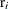as having its own probability of success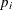, and the likelihood is as follows: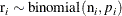You can use the logit link function to link the covariates of each observation, seed and extract, to the probability of success,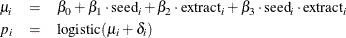where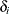is assumed to be an iid random effect with a normal prior: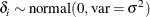The fourregression coefficients and the standard deviation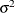in the random effects are model parameters; they are given noninformative priors as follows: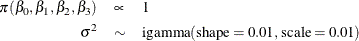Another way of expressing the same model is as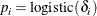where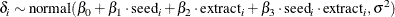The two models are equivalent. In the first model, the random effectscenters at 0 in the normal distribution, and in the second model,centers at the regression mean. This hierarchical centering can sometimes improve mixing.

The following statements fit the second model and generate Output 61.7.1:

proc mcmc data=seeds outpost=postout seed=332786 nmc=20000;
ods select PostSumInt;
parms beta0 0 beta1 0 beta2 0 beta3 0 s2 1;
prior s2 ~ igamma(0.01, s=0.01);
prior beta: ~ general(0);
w = beta0 + beta1*seed + beta2*extract + beta3*seed*extract;
random delta ~ normal(w, var=s2) subject=ind;
pi = logistic(delta);
model r ~ binomial(n = n, p = pi);
run;


The PROC MCMC statement specifies the input and output data sets, sets a seed for the random number generator, and requests a large simulation size. The ODS SELECT statement displays the summary statistics and interval statistics tables. The PARMS statement declares the model parameters, and the PRIOR statements specify the prior distributions forand.

The symbol w calculates the regression mean, and the RANDOM statement specifies the random effect, with a normal prior distribution, centered at w with variance. Note that the variable w is a function of the input data set variables. You can use data set variable in constructing the hyperparameters of the random-effects parameters, as long as the hyperparameters remain constant within each subject group. The SUBJECT= option indicates the group index for the random-effects parameters.

The symbol pi is the logit transformation. The MODEL specifies the response variable r as a binomial distribution with parameters n and pi.

Output 61.7.1 lists the posterior mean and interval estimates of the regression parameters.

Output 61.7.1: Logistic Regression Random-Effects Model

 Logistic Regression Random-Effects Model

The MCMC Procedure

Posterior Summaries and Intervals
Parameter N Mean Standard
Deviation
95% HPD Interval
beta0 20000 -0.5570 0.1929 -0.9422 -0.1816
beta1 20000 0.0776 0.3276 -0.5690 0.7499
beta2 20000 1.3667 0.2923 0.8463 1.9724
beta3 20000 -0.8469 0.4718 -1.7741 0.0742
s2 20000 0.1171 0.0993 0.00163 0.3045